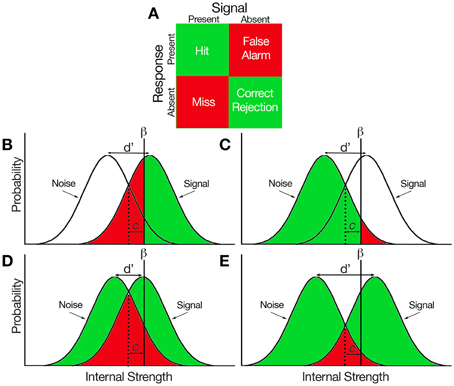# Compute Signal Detection Theory Indices with R

March 28, 2018
By

Want to share your content on R-bloggers? click here if you have a blog, or here if you don't.

## Signal Detection Theory Indices (dprime, beta…)

Signal detection theory (SDT) is used when psychologists want to measure the way we make decisions under conditions of uncertainty. SDT assumes that the decision maker is not a passive receiver of information, but an active decision-maker who makes difficult perceptual judgments under conditions of uncertainty. To apply signal detection theory to a data set where stimuli were either present or absent, and the observer categorized each trial as having the stimulus present or absent, the trials are sorted into one of four categories: Hit, Miss, Correct Rejection and False Alarm.Based on the proportions of these types of trials, we can compute indices of sensitivity and response bias:

• d’ (d prime): The sensitivity. Reflects the distance between the two distributions: signal, and signal+noise and corresponds to the Z value of the hit-rate minus that of the false-alarm rate.
• beta: The bias (criterion). The value for beta is the ratio of the normal density functions at the criterion of the Z values used in the computation of d’. This reflects an observer’s bias to say ‘yes’ or ‘no’ with the unbiased observer having a value around 1.0. As the bias to say ‘yes’ increases (liberal), resulting in a higher hit-rate and false-alarm-rate, beta approaches 0.0. As the bias to say ‘no’ increases (conservative), resulting in a lower hit-rate and false-alarm rate, beta increases over 1.0 on an open-ended scale.
• A’ (aprime): Non-parametric estimate of discriminability. An A’ near 1.0 indicates good discriminability, while a value near 0.5 means chance performance.
• B’‘D (b prime prime d): Non-parametric estimate of bias. A B’‘D equal to 0.0 indicates no bias, positive numbers represent conservative bias (i.e., a tendency to answer ‘no’), negative numbers represent liberal bias (i.e. a tendency to answer ‘yes’). The maximum absolute value is 1.0.
• c: Another index of bias. the number of standard deviations from the midpoint between these two distributions, i.e., a measure on a continuum from “conservative” to “liberal”.

To compute them with `psycho`, simply run the following:

``````library(psycho)

# Let's simulate three participants with different results at a perceptual detection task
df <- data.frame(Participant = c("A", "B", "C"),
n_hit = c(1, 2, 5),
n_fa = c(1, 3, 5),
n_miss = c(6, 8, 1),
n_cr = c(4, 8, 9))

indices <- psycho::dprime(df\$n_hit, df\$n_fa, df\$n_miss, df\$n_cr)
df <- cbind(df, indices)
``````
Participant n_hit n_fa n_miss n_cr dprime beta aprime bppd c
A 1 1 6 4 -0.1925836 0.8518485 0.5000000 0.9459459 0.8326077
B 2 3 8 8 -0.1981923 0.8735807 0.4106061 0.8285714 0.6819377
C 5 5 1 9 0.9952151 0.8827453 0.5000000 -0.9230769 -0.1253182

R-bloggers.com offers daily e-mail updates about R news and tutorials about learning R and many other topics. Click here if you're looking to post or find an R/data-science job.
Want to share your content on R-bloggers? click here if you have a blog, or here if you don't.